# Algebra - math word problems

#### Number of problems found: 2638

• Two runners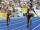Two runners ran simultaneously towards each other from locations distant 34.6 km. The average speed of the first runner was 1/5 higher than the average speed of the second runner. How long should each ran a 34.6 km, if you know that they meet after 67 m
• EquationEquation ? has one root x1 = 8. Determine the coefficient b and the second root x2.
• Machines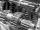In the workshop are three machines. The first is treated 250 parts for 4.7 hours, the second is treated 300 parts for 1.9 hours and the third is treated 230 parts for 4.6 hours. How long will take to make 3100 parts if worked all three machines at the
• Rectangles - sidesOne side of the rectangle is 10 cm longer than second. Shortens longer side by 6 cm and extend shorter by 14 cm increases the area of the rectangle by 130 cm2. What are the dimensions of the original rectangle?
• Two valves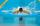Water fill the pool by two valves for 11 days. After 7 days the first valve was stopped and the second valve fill pool for 7 days. How many days took to fill the pool each of the valves individually?
• FractionsSort fractions ? by its size. Result write as three serial numbers 1,2,3.
• Rectangle - sidesWhat is the perimeter of a rectangle with area 266 cm2 if length of the shorter side is 5 cm shorter than the length of the longer side?
• Pedestrian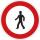Pedestrian came started at 8h in the morning with speed 4.4 km/h. At half-past eleven cyclist started at 26 km/h same way. How many minutes take cyclist to catch up pedestrian?
• Parquet floor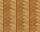Three workers laid parquet floor for 2.301 hour. The first one would do this work alone 7 hours, the second in 6 hours. For how many hours would fulfill this work the third worker, if he worked alone?
• Painters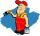First painter painted fence himself for 4 hours, the second painter 1.2 hours sooner than first, and the third painters slowest up to 16 hours. How long took them paint fence if worked together?
• Square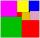If we increase one side of square by its one-half then square perimeter increase by 10 cm. What is the side of the square?
• Bottle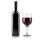Bottle with wine costs 58 USD. Wine is 27 USD more expensive than the empty bottle. How much is the bottle itself?
• Swimming poolThe pool shape of cuboid is 299 m3 full of water. Determine the dimensions of its bottom if water depth is 282 cm and one bottom dimension is 4.7 m greater than the second.
• ParabolaFind the equation of a parabola that contains the points at A[6; -5], B[14; 9], C[23; 6]. (use y = ax2+bx+c)
• Coffee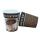Coffee from the machine in the cup cost 28 cents. Coffee is 20 cents more expensive than the cup. How much is the cup?
• 7 triangle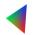The triangle area is 26.7 cm2. Determine the side length l if appropriate height hl = 45.3 cm.
• PopulationThe town has 65,000 inhabitants. 40 years ago there were 157,000. How many people will live in town in 10 years if the average rate in population is as in previous years?
• Drive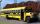At the bus stop 8 people take out and 10 people take into bus. Next stop 6 take out and 5 take in. On the third stop 6 take out and 3 take in. The bus traveled further with 39 people. How many passengers were originally at the bus?
• Linear independence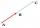Determine if vectors u=(-4; -5) and v=(20; 25) are linear Linear dependent.
• Column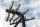Perpendicular pole high 8 m tall broke and its toe fell 2.7 m from the bottom of the pole. At what height above the ground pole broke?

Do you have an interesting mathematical word problem that you can't solve it? Submit a math problem, and we can try to solve it.

We will send a solution to your e-mail address. Solved examples are also published here. Please enter the e-mail correctly and check whether you don't have a full mailbox.

Please do not submit problems from current active competitions such as Mathematical Olympiad, correspondence seminars etc...